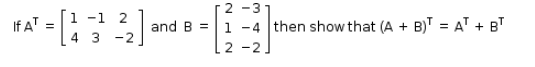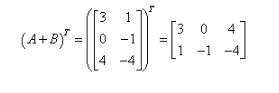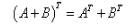# Given A^T and B matrix Then show that (A+B)^T=A^T+B^T

Given A and B matrixTherefore,andThus,